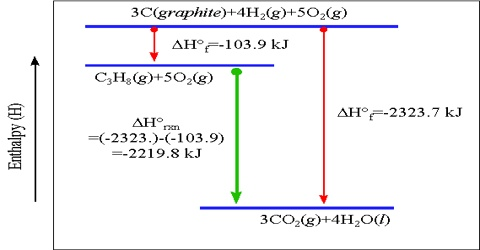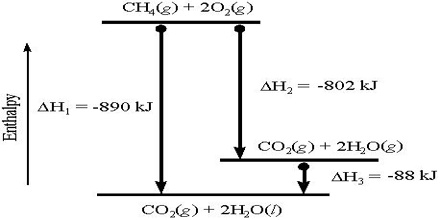# Heat of Reaction or Enthalpy of Reaction

Heat of Reaction or Enthalpy of Reaction

The Heat of Reaction (also known Enthalpy of Reaction) is the change in the enthalpy of chemical reaction that happens at a constant pressure. The enthalpy of reaction is ordinarily defined as the amount of heat evolved or absorbed when the reaction has taken place at constant pressure between the numbers of moles of the reactants as shown in the balanced equation.

If heat is evolved the reaction is said to be exothermic and when heat is absorbed the reaction is an endothermic one. Heat of reaction may also be defined as the difference in enthalpy or heat content, ∆H, between the products and the reactants. It is a thermodynamic unit of measurement useful for calculating the amount of energy per mole either released or produced in a reaction. The amount of heat change is expressed in kilojoule (KJ).The following examples will, perhaps, clarify the definition;

(a) C(s) + O2(g) → CO2 (g); ∆H0 = – 393.5 KJ

(b) 2 C(s) + 2H2 (g) → C2H4 (g); ∆H0 = + 52.3 KJ

(a) When one mole (12g) of graphite reacts with one mole (32g) of gaseous oxygen to produce one mole (44g) of gaseous Carbon dioxide, the heat of reaction or the change in heat content is -393.5 KJ. Here the heat content of CO2 (g) is less than the sum of the heat contents of C(s) and O2 (g) and heat is evolved, the reaction is exothermic and the heat of reaction, ∆H0; in negative.

(b) In this reaction two moles (24 g) of graphite combine with two moles (4 g) of hydrogen to form one mole (28 g) of ethylene and the heat of reaction is + 52.3 KJ. The heat content of one mole of C2H4 (g) is more than the sum of the heat contents of 2 moles of C(s) and 2 moles of H2 (g) and heat is absorbed; the reaction is endothermic and the heat of reaction, ∆H0, is positive.